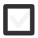# Draw a FIGURE, a line of symmetry, & how the figure is mirrored. Draw a rotationally symmetrical figure.+1 vote
117 views
In 10-12
edited

## 1 lesson

+1 vote
by Guru (6.1k)
selected

Best lesson

Rotational Symmetry

Let’s learn the theory:

Exercises:

2. What are the coordinates when the following points are mirrored according to the x-axis?

• (4, 1)
• (0, -5)
• (-6, 0)
• (-4, -7)

3. What are the coordinates when the above points are mirrored according to the y-axis?

4. Draw a triangle A(-1, 3), B(-2, 0) and C(2, -1). Mirror the triangle by the point (0, 1), What are the vertices of the mirrored triangle?